Refer to our Texas Go Math Grade 7 Answer Key Pdf to score good marks in the exams. Test yourself by practicing the problems from Texas Go Math Grade 7 Module 14 Quiz Answer Key.

14.1 Personal Budgets

For 1-3, use the following information on the Plimpton family’s monthly budget.

Their net monthly income is $4,500. They have an emergency fund that is 6% of their monthly budget and they have a savings of 7% per month. Medical costs are$675 per month, housing is $1,485, and transportation costs$450. Food accounts for 17% of the monthly budget and they spend 5% on entertainment. They also spend 7% of the budget on clothing each month.

Question 1.
How much does the Plimpton family spend on clothing each month? ____
Net monthly income of Plimpton family = $4, 500 Percentage of budget used for clothing 7% Amount spent on clothing = $$\frac{\text { Percentage }}{100}$$ × Net monthly income Amount spent on clothing = $$\frac{7}{100}$$ × 4,500 = 0.07 × 4,500 =$315
Hence, Plimpton family spend $315 monthly on cLothing. Question 2. How much does the Plimpton family spend on entertainment each year? ____ Answer: Net monthly income of Plimpton family =$4, 500
Percentage of budget used for Entertainment = 5%
Amount spent on Entertainment = $$\frac{\text { Percentage }}{100}$$ × Net monthly income
Amount spent on Entertainment = $$\frac{5}{100}$$ × 4, 500
= 0.05 × 4, 500
= $225 Hence, Plimpton family spend$225 monthly on Entertainment.

Question 3.
How much more does the Plimpton family spend on food than on savings each month? ____
Net monthly income of Plimpton family = $4, 500 Percentage of budget used for food = 17% Percentage of budget used for saving = 7% Amount spent on food = $$\frac{\text { Percentage }}{100}$$ × Net monthly income Amount spent on food = $$\frac{17}{100}$$ × 4, 500 = 0.17 × 4,500 =$765
Amount spent on saving = $$\frac{7}{100}$$ × 4, 500
= 0.07 × 4,500
= $315 Hence, Plimpton family spend$765 monthly on food and $315 is saving. Question 4. What percent of the net monthly income goes to housing? ____ Answer: Net monthly income of Plimpton family =$4, 500
Amount from budget that goes to housing = $1,485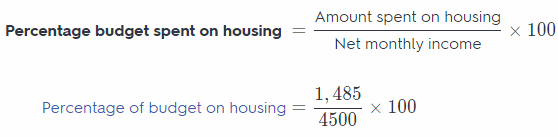Percentage of budget on housing = $$\frac{1,485}{4500}$$ × 100 = 0.33 × 4,500 = 33% Hence, Plimpton family spend 33% of monthly budget on housing. 14.2 Planning a Budget Question 5. Ms. Wofford and her two sons live in Lubbock, Texas, where she makes$3,200 a month. She is thinking of transferring to Abilene and uses an online family budget estimator to see her potential monthly expenses. Use the table below. Is a transfer to Abilene a good financial move for the Woffords? Explain.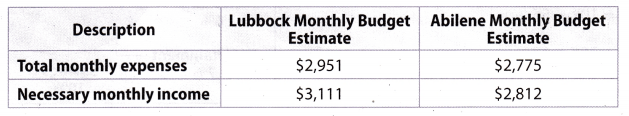Minimum monthly saving of Ms. Wofford in Lubbock = 3,111 – 2, 951 = $160 Minimum monthly saving of Ms. Wofford in Abilene = 2,812 – 2,775 =$37
Now on comparing the minimum monthly saving in both the city, we can see that saving in the Lubbock is more as
compared to the saving in Abilene. So for Ms. Wofford transfer to Abilene will, be not a good financial move.
Hence, transfer to Abilene will be not good move for Ms. Wofford.

14.3 Constructing a Net Worth Statement

Question 6.
Calvin lives in a home with a value of $185,000 and has a mortgage of$ 145,000. He has a stock portfolio worth $11,700. He owns his car, which is valued at$10,500. He has $15,300 in student loans to repay. He has a credit card balance of$6,228. He also has $3,400 in a bank account. What is Calvin’s net worth? ____ Answer: Net worth = Value of assets – Value of Liabilities =$210,600 – $166,528 =$44,072
Hence, Calvin’s net worth is $44 072 Texas Go Math Grade 7 Module 14 Mixed Review Texas Test Prep Answer Key Selected Response Question 1. Which of the following is an example of income? (A) insurance (B) emergency savings (C) wages (D) taxes Answer: (C) wages Wages are the amount which anyone receives when he/she completes the work. So wages is an income for any anyone. Question 2. Which of the following is an example of a variable expense? (A) weekly martial arts lesson (B) rent (C) entertainment (D) monthly bus pass Answer: (C) entertainment Variable expenses are the expense which varies over the time. Variable expanse is never fixed and depends on the daily life of persons. Example of variable expense are snacks, entertainment, etc. Question 3. A boating company on the lake charges a$10 equipment fee and $5.50 per hour to rent a canoe. Write the equation of the linear relationship. (A) y = 10x + 5.5 (B) y = 10x + 55 (C) y = 55x + 10 (D) y = 5.5x + 10 Answer: (D) y = 5.5x + 10 Equipment fee of boating company =$10
Per hour charge for renting canoe = $5.50 Renting charge of canoe for x hours = 5.5 × x Total cost of renting canoe for x hours = Equipment fee + Renting fee for x hours. In linear equation form : y = 10 + 5.5x Hence, Option D is correct answers. Question 4. Barry owns a home with a value of$170,000. He owes $4,400 on his cat, which is valued at$11,500. He has $9,500 in student loans to repay. He owns$3,300 worth of musical equipment. He has a credit card balance of $2,117. He also has$2,900 in a bank account. What is Barry’s net worth?
(A) $157,483 (B)$171,683
(C) $165,883 (D)$175,917
(B) $171,683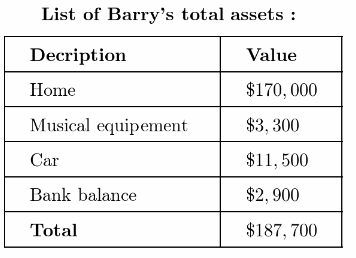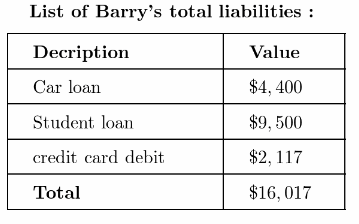Net worth = Value of assets – Value of Liabilities =$187,700 – $16,017 =$171,683

Question 5.
Which of the following is an example of an asset?
(A) car loan
(B) credit card bill
(C) savings bond
(D) rent
(C) savings bond
Assets are something which we own and it has some positive cash value which means we will get some money
when we will sell it. Savings bond are issued by the government and when we will sell it back to the government
after certain duration of time we will get money back with additional some amount of interest.

Gridded Response

Question 6.
The Garza family consists of two adults and two children. Their current monthly income is $4,800. The circle graph shows their monthly budget. How much money in dollars do the Garzas spend on housing each month?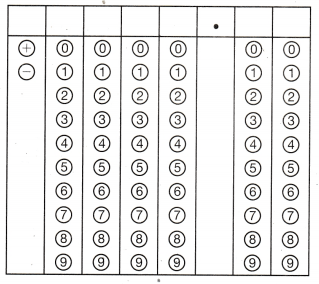Answer: Current monthly income of Garza family =$4, 800
Percentage of budget used for housing = 30%
Amount spent on housing = $$\frac{\text { Percentage }}{100}$$ × Net monthly income
Amount spent on housing = $$\frac{30}{100}$$ × 4, 800
= 0.3 × 4,800
= $1440 Hence, Garza family spend$1440 monthly on housing.

Scroll to Top